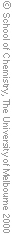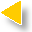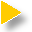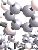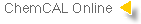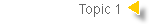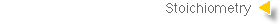##### Review
Having completed this tutorial you should be able to :Be able to recall the common prefixes to denote the 109, 106,103,10-1,10-2,10-3,10-6,10-9 and10-12 multipliers for units and be able to convert between various decimal scales for units.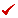Be aware of the importance of significant figures in calculations and use them appropriatelyUse atomic and molar masses and the Avogadro Number, NA, to calculate and interconvert masses, numbers of atoms or molecules and molar quantities.Balance chemical equations.Calculate yields of chemical processes based on their equations and the use of molar quantities.Use and interconvert masses to calculate molar concentrations of substances.